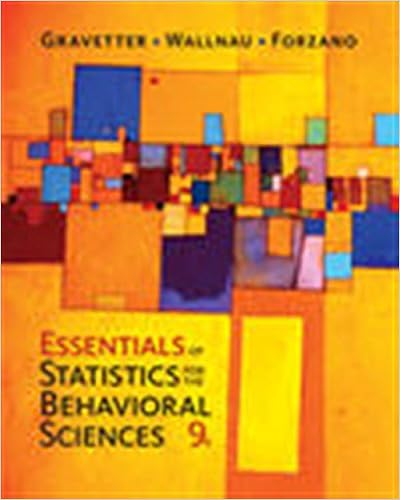# There either is or is not an effect and we need to

• 50
• 100% (5) 5 out of 5 people found this document helpful

This preview shows page 37 - 39 out of 50 pages.

##### We have textbook solutions for you!
The document you are viewing contains questions related to this textbook.The document you are viewing contains questions related to this textbook.
Chapter 8 / Exercise 22
Essentials of Statistics for the Behavioral Sciences
Gravetter/WallnauExpert Verified
There either is or is not an effect and we need to decide, based on our statistics, which one is more likely to be true. o Ex. Aggression modelling experiment Null hypothesis H 0 – the population mean of the model group is equal to the population mean of the no-model group Research hypothesis H 1 – the population mean of the model group is not equal to the population mean of the no-model group
##### We have textbook solutions for you!
The document you are viewing contains questions related to this textbook.The document you are viewing contains questions related to this textbook.
Chapter 8 / Exercise 22
Essentials of Statistics for the Behavioral Sciences
Gravetter/WallnauExpert Verified
- The null hypothesis is used in inferential statistics because it is a very precise statement – the population means are exactly equal o This precision permits us to know the probability of the outcome of the study occurring if the null hypothesis is true in the population o Such precision is not possible with the research hypothesis, so we infer that the research hypothesis is likely true in the population only by rejecting the null hypothesis o Null hypothesis is rejected when there is a very low probability that the obtained results could be due to random error This is what is meant by statistical significance: A significant result is one that has a very low probability of occurring if there is no effect in the population (e.g. group means are equal) Significance indicates that there is a low probability that the difference between the obtained sample means (or the non-zero correlation) was due to random error. PROBABILITY AND SAMPLING DISTRIBUTIONS - Probability is the likelihood of the occurrence of some event or outcome. - Probability in statistical inference is used in much the same way o We want to specify the probability that an event (in this case, a difference between means in the sample) will occur if there is no difference in the population PROBABILITY: THE CASE OF MIND READING - Ex. Friend claims to have mind reading or ESP, you decide to test by flipping coins 10 times o Goal is to determine whether answers reflect random error (guessing) or whether something more than random error is occurring o Null hypothesis is that only random error is operating o Research hypothesis sis that the number of correct answers shows more than random or chance guessing - The probability required for significance is called the Alpha Level o Most common alpha level probability used is 0.05 Outcome is considered significant when there is a 0.05 or less probability of obtaining the results if the null hypothesis is actually true. If it is very unlikely that random error is responsible for the obtained results, the null hypothesis is rejected. SAMPLING DISTRIBUTIONS - A probability distribution called Binomial distribution - Such distributions are called null hypothesis sampling distributions or simply sampling distributions - Sampling distribution is based on the assumption that the null hypothesis is true; o in the mind reading example, the null hypothesis is that the person is only guessing and should therefore get 50% correct.
•••